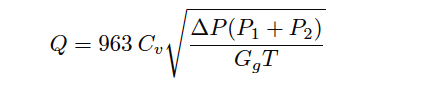# Gas Valve Sizing

Sizing a control valve for gas or vapor service is more complicated than for liquid service, due to the compressibility of gases and vapors.

As a gas or vapor compresses with changes in pressure, its density changes correspondingly. In previous mathematical analyses of fluid flow restriction, one of our assumptions was that fluid density (ρ) remained constant.

This assumption may hold true for some flowing gas conditions as well, provided minimal pressure changes within the path of flow.

However, for most gas control valve applications where the very purpose of the valve is to introduce substantial pressure changes, the assumption of constant gas density is unrealistic.

## Gas Valve SizingShown here is one of the simpler gas valve sizing equations you will encounter:Where,

Q = Gas flow rate, in units of Standard Cubic Feet per Hour (SCFH)
Cv = Valve capacity coefficient
ΔP = Pressure dropped across valve, pounds per square inch differential (PSID)
P1 = Upstream valve pressure, pounds per square inch absolute (PSIA)
P2 = Downstream valve pressure, pounds per square inch absolute (PSIA)
Gg = Specific gravity of gas (ratio of gas density to standard air density)
T = Absolute temperature of gas in degrees Rankine (oR), equal to degrees Fahrenheit plus 459.67

This equation holds true only for “subcritical” flow, where the moving gas stream velocity never approaches the speed of sound (Note). Other equations exist for calculating flow rates of gas through control valves in the presence of sonic flow regimes.

Note the inclusion of absolute pressures in this equation, and not just differential pressure (ΔP, or P1 − P2). This is intended to correct for effects related to compression of the gas under pressure.

Note : The ISA Handbook of Control Valves cites this equation as being valid for conditions where the valve’s downstream pressure (P2) is equal to or greater than one-half the upstream pressure (P1), with both pressures expressed in absolute units. In other words, P2 ≥ 0.5P1 or P1 ≤ 2P2. An upstream:downstream pressure ratio in excess of 2:1 usually means flow through a valve will become choked.

Valve sizing is complicated enough, both for liquid and gas service, that the use of valve sizing computer software is strongly recommended as opposed to hand-calculations.

The number of important parameters, nonlinear factors, and alternative equations relevant to control valve sizing are numerous enough to bewilder most technicians (and more than a few engineers).

Valve sizing software will also predict noise levels generated by the valve, and in many cases specify actual valve trim styles offered by the manufacturer for mitigating problems such as noise.

Also Read : Basics of Control Valve Sizing